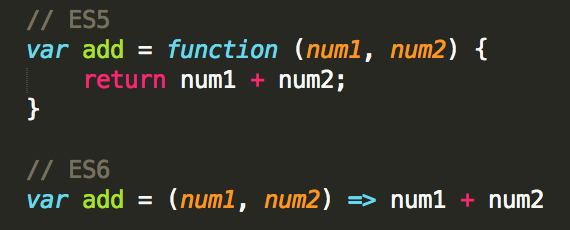# Understanding the Arrow function ( in javascript )Why was the arrow function created ?
As you may know that the arrow function was added to ES6.
To solve two things.
1. Shorter function declarations
2. to solve the “this” problem

1. Shorter function is self evident:

Lets take simple array manipulation.

The map() method creates a new array with the results of calling a provided function on every element in the calling array

Example A: Let us find the sqaure root of a given list of numbers

```var listofNumbers = [4,9,25];
console.log(listofNumbers.map(Math.sqrt));
//[2,3,5]```

In fact to lessen any possible confusion for the novices, we can even rewrite example A into something more familliar :

Example B:

```var listofNumbers = [4,9,25];
console.log( listofNumbers.map( function(number){
return Math.sqrt(number);
}) );
// [2,3,5]```

Now lets say we want to find the squares of a given list of numbers.
We can do this via the Math.pow() function. Here is where it gets tricky.

Unlike the Math.sqrt function that used in Example A. we can’t do that for the Math.pow() method ? Why because Math.pow(base,exponent), we need to specify both base and exponent.

We can do this ofcourse by using anonymous functions.
Example C

```var listofNumbers = [2,3,5];
console.log( listofNumbers.map( function(number){
return Math.pow(number,2);
}) );
// [4,9,25]```

Now how do we right this using arrow function in ES6

Example D:

`console.log( listofNumbers.map( (number) => Math.pow(number,2) ) );`

As you can see example D is a lot shorter than Example C:

Simillarly lets say we have a list of names = [‘ram’,’hari’,’shyam’] and we have to find how many characters each name is:
ES5 without arrow function
Example E:

```var names = ['ram','hari','shyam'];
console.log( names.map(function(name){
return name.length
}) );```

Now using arrow function this becomes:
Example F:

```var names = ['ram','hari','shyam'];
console.log( names.map( name => name.length) );
//can also be written as
//notice the paranthesis
console.log( names.map((name) => {
return name.length;
}));```

Basically what’s happening is not much has changed, the syntax for arrow function is
(param1,param2…param(n)) => {
// do something
}

compare that to ES5 function code
function (param1,param2…param(n)){
// do something
}

So basically what happens is the function keyword is replaced and placed after the two opening and closing paranthesis that’s it.
Also the paranthesis is not required if there is only one parameter. hence the name => name.length

## One thought on “Understanding the Arrow function ( in javascript )”

1.Biraj Bajracharya says:

Awesome help.

This site uses Akismet to reduce spam. Learn how your comment data is processed.